ISEE Lower Level Math : Ratio and Proportion

Example Questions

1 2 3 4 6 Next →

Example Question #51 : Ratio And Proportion

On a typical day, twenty percent of the pies that a pie store sells are blueberry pies. If the store sells forty pies on just such a typical day, how many blueberry pies were likely sold?Explanation:

If  store sellspies, and typicallyof the pies that are sold are blueberry, that means thatof the pies sold were probably blueberry.

Given thatofis, it follows thatofisTherefore,is the correct answer.

Example Question #52 : Ratio And Proportion

The ratio of rabbits to guinea pigs to hamsters in a pet store is 3:2:4. If there are 12 rabbits in the pet store, how many hamsters are there?Explanation:

Given that the ratio of rabbits to guinea pigs to hamsters in a pet store is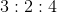, each number should be 4 times greater if there are 12 rabbits. This is because 3 times 4 is 12.

Therefore, we can transform the of rabbits to guinea pigs to hamsters into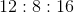Therefore, there are 16 hamsters.

Example Question #53 : Ratio And Proportion

Reduce the following proportion to its simplest form: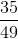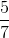Explanation:

To simplify, you find a common factor for both the numerator and denominator and divide them both by that value.

Bothandhave a common factor of.

This gives us,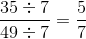.

Example Question #54 : Ratio And Proportion

Rachel and Michael earn the same amount of money per hour.  Rachel earns $64.00 for eight hours of work. How long would it take Michael to earn$96.00?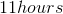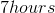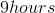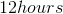Explanation:

In order to solve this problem, you would set up a direct proportion: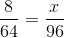To make the process even simpler, you could first reduce the first fraction in this direct proportion. The fraction was reduced to simplest form by dividing both the numerator and the denominator by 8, the greatest common factor or the GCF.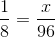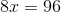Divide both sides by the coefficient of x, which is 8: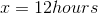Example Question #55 : Ratio And Proportion

On a map, one inch is equal tomiles. How many inches will be on the map for a a distance of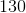miles?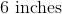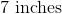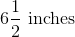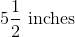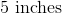Explanation:

The proportion for the problem will be,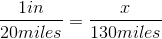.

You then cross multiple to get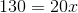.

To find, you divide both sides byto get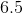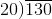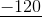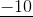Therefore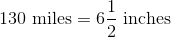1 2 3 4 6 Next →